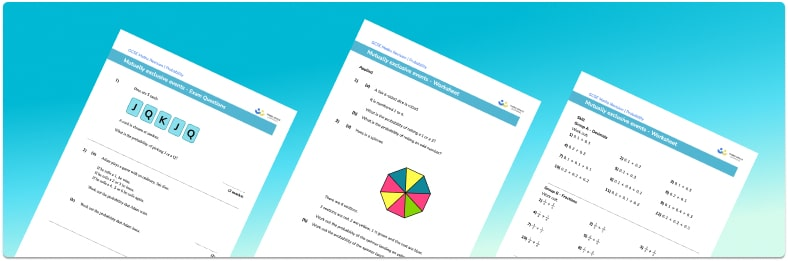# Mutually Exclusive Events Worksheet• Section 1 of the mutually exclusive events worksheet contains 20+ skills-based mutually exclusive events questions, in 3 groups to support differentiation
• Section 2 contains 4 applied mutually exclusive events questions with a mix of worded problems and deeper problem solving questions
• Section 3 contains 3 foundation and higher level GCSE exam style mutually exclusive events questions
• Answers and a mark scheme for all mutually exclusive events questions are provided
• Questions follow variation theory with plenty of opportunities for students to work independently at their own level
• All questions created by fully qualified expert secondary maths teachers

Suitable for GCSE maths revision for AQA, OCR and Edexcel exam boards

• This field is for validation purposes and should be left unchanged.

You can unsubscribe at any time (each email we send will contain an easy way to unsubscribe). To find out more about how we use your data, see our privacy policy.

### Mutually exclusive events at a glance

Mutually exclusive events are events that cannot occur at the same time, for example rolling a 1 and a 2 on a dice. For two mutually exclusive events A and B, the probability of an event A happening OR an event B happening, known as P(A or B), is equal to the sum of the probability of event A happening P(A), added to the probability of event B happening (P(B).

In mathematical notation: P(A or B) = P(A) + P(B).

Let’s consider a pack of playing cards. The probability of getting a face card is 12 out of 52, and the probability of getting a card showing a prime number is 16 out of 52. The probability of getting a face card or a prime number is the sum of these two probabilities, which is 28 out of 52. The sum of the probabilities of an exhaustive set of mutually exclusive events is 1.

An example of a non-mutually exclusive event would be choosing a face card or a red card. Half of the face cards are red cards and so we add an extra condition by removing any overlapping outcomes (the probability of a face card AND a red card) from the probability of the two events happening. As 12 cards are face cards, 26 cards are red, and 6 cards are a face card and a red card, the number of cards that are face cards or red cards is 12 + 26 – 6 = 32, so P(face card or red) = 32 out of 52.

In mathematical notation: P(A or B) = P(A) + P(B) – P(A and B)

To find the probability of an event not happening, we subtract the probability of it happening from 1. If the probability of getting on odd number when rolling a biased dice is 3 out of 4 or 0.75, then the probability of getting an even number (i.e. not getting an odd number) is 1 – 0.75 = 0.25.

Independent events are events where the outcome of one event does not affect the outcome of the other. For independent events P(A and B)=P(A) x P(B). When considering compound events, we often represent the different outcomes on a probability tree diagram to help us visualise the options. This is also useful when we find probabilities of dependent events.

Looking forward, students can then progress to additional probability worksheets, for example the combined events probability worksheet, the conditional probability worksheet, or the describing probabilities worksheet.For more teaching and learning support on probability our GCSE maths lessons provide step by step support for all GCSE maths concepts.

## Do you have KS4 students who need more focused attention to succeed at GCSE?There will be students in your class who require individual attention to help them succeed in their maths GCSEs. In a class of 30, it’s not always easy to provide.

Help your students feel confident with exam-style questions and the strategies they’ll need to answer them correctly with our dedicated GCSE maths revision programme.

Lessons are selected to provide support where each student needs it most, and specially-trained GCSE maths tutors adapt the pitch and pace of each lesson. This ensures a personalised revision programme that raises grades and boosts confidence.

Find out more